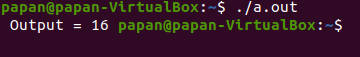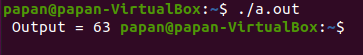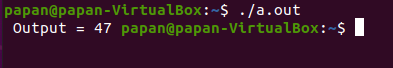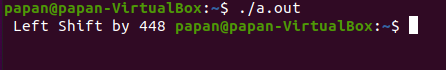| by Arround The Web | No comments

## Bitwise Operator in C

In C language Operator Groups are present. There are seven types of operators present. They are:

1. Unary
2. Arithmetic
3. Bitwise
4. Relational
5. Logical
6. Conditional
7. Assignment

In C there is a preceding rule that exists in case of operator Groups. If in a problem there are multiple operators present, then this type of problem is solved according to this order of operator groups.

Bitwise operator is the member of this operator groups. There are many types of relational operators present in C language.

There are six types of bitwise operator:

1. Bitwise AND ( & )
2. Bitwise OR ( | )
3. Bitwise XOR ^ ( exclusive OR )
4. Bitwise NOT ~ ( world’s complement )
5. Right Shift >>
6. Left Shift <<

## Bitwise AND ( & ) operator:

0 & 0 = 0

0 & 1 = 0

1 & 0 = 0

1 & 1 = 1

Example:

int x ;

x = 23 & 56 ;

23 = 0000 0000 0001 0111 ( In Binary )

56 = 0000 0000 0011 1000 ( In Binary )

16 = 0000 0000 0001 0000

Integer constant consumed in DOS based architecture 2 bytes.

## Programming Example 1:

#include <stdio.h>

int main()
{
int x;
x= 23 &56;
printf(" Output = %d ", x);
return 0;
}

Output:### Explanation:

Here is an example of bitwise and ( & ) operator. Bitwise and operator acts as a multiplication operator. The given expression is:

x = 23 & 56;

Here we give an expression, 23 & 56. Bitwise and operator convert both the inputs 23 and 56 to the binary values. Then multiply those values. The result is 16.

## Bitwise OR operator:

0 | 0 = 0

0 | 1 = 1

1 | 0 = 1

1 | 1 = 1

### Example:

int x ;

x = 23 | 56;

23 = 0000 0000 0001 0111 (In Binary)

56 = 0000 0000 0011 1000 (In Binary)

63 = 0000 0000 0011 1111

## Programming Example 2:

#include <stdio.h>

int main()
{

int x;
x= 23 | 56;
printf(" Output = %d ", x);
return 0;
}

Output:### Explanation:

Here is an example of bitwise or ( ! ) operator. Bitwise or operator acts as a addition operator. The given expression is:

x= 23 & 56 ;

Here is an expression, 23 ! 56. Bitwise and operator convert both the inputs 23 and 56 to the binary values. Then sum those values. The result is 63.

## Bitwise XOR operator:

0 ^ 0 = 0

0 ^ 1 = 1

1 ^ 0 = 1

1 ^ 1 = 0

### Example:

int x ;

x = 23 ^ 56;

23 = 0000 0000 0001 0111 ( In Binary )

56 = 0000 0000 0011 1000 ( In Binary )

47 = 0000 0000 0010 1111

## Programming Example 3:

#include <stdio.h>

int main()
{
int x;
x= 23 ^ 56;

printf(" Output = %d ", x);
return 0;
}

Output:### Explanation:

Here is an example of bitwise XOR ( ^ ) operator. Bitwise XOR operator acts if both the inputs are same ( 0 or 1 ), the result will be zero ( 0 ). If both inputs are different (either 0 or 1), then the result will be one ( 1 ). The given expression is:

x = 23 & 56 ;

Here is an expression, 23 ^ 56. Bitwise and operator convert both the inputs 23 and 56 to the binary values. The result is 47.

### Right Shift:

int x ;

x = 56 >>2;

56 = 0000 0000 0011 1000

14 = 0000 0000 0000 1110

In Right Shift operator when any number is given >> 2, it means we must add 2 zero, >> 3 add 3 zero, on the left side of binary number that is given (56), total 16 bit exist, so, the right most 2 digit (here 00) are removed.

## Programming Example 4:

#include <stdio.h>

int main()
{
int x;
x= 56 >>2 ;
printf("Right Shift by %d ", x);
return 0;
}

Output:### Explanation:

Here is an example of bitwise right shift >> operator. Bitwise right shift operator acts as a place shifted operator. The given expression is:

x = 23 >> 56 ;

Here is an expression, 23 ! 56. Bitwise and operator convert both the inputs 23 and 56 to the binary values. The result is 14.

### Left Shift:

int x;

x = 56 <<3 ;

56= 0000 0000 0011 1000

448=0000 0001 1100 0000

In Left Shift, operator when any number given << 3 to add 3 zeros on the right corner of the binary number that is given (56) here, total 16 bits exist, so the left most 3 digit (here 000) are removed.

## Programming Example 5:

#include <stdio.h>

int main()
{
int x;
x= 56 <<3 ;
printf(" Left Shift by %d ", x);
return 0;
}

Output:### Explanation:

Here is an example of bitwise Left shift ( << ) operator. Bitwise or operator acts as a place shifted operator. The given expression is:

x = 23 << 56 ;

Here we give an expression, 23 << 56. Bitwise and operator actually convert both the inputs 23 and 56 to the binary values. The result is 448.

## Conclusion:

From the above discussion about the concept of bitwise operator, we see different types of programming example of bitwise operator: How bitwise operator works or what will be its output we discuss here. Basically, bitwise operators give us an output on the base of binary values. Bitwise operator gives the programmer a variation of calculating different types of binary numbers.

Source: linuxhint.com